Travis-CI:# matplotlib.pyplot.subplot¶

`matplotlib.pyplot.``subplot`(*args, **kwargs)

Return a subplot axes positioned by the given grid definition.

Typical call signature:

```subplot(nrows, ncols, plot_number)
```

Where nrows and ncols are used to notionally split the figure into `nrows * ncols` sub-axes, and plot_number is used to identify the particular subplot that this function is to create within the notional grid. plot_number starts at 1, increments across rows first and has a maximum of `nrows * ncols`.

In the case when nrows, ncols and plot_number are all less than 10, a convenience exists, such that the a 3 digit number can be given instead, where the hundreds represent nrows, the tens represent ncols and the units represent plot_number. For instance:

```subplot(211)
```

produces a subaxes in a figure which represents the top plot (i.e. the first) in a 2 row by 1 column notional grid (no grid actually exists, but conceptually this is how the returned subplot has been positioned).

Note

Creating a subplot will delete any pre-existing subplot that overlaps with it beyond sharing a boundary:

```import matplotlib.pyplot as plt
# plot a line, implicitly creating a subplot(111)
plt.plot([1,2,3])
# now create a subplot which represents the top plot of a grid
# with 2 rows and 1 column. Since this subplot will overlap the
# first, the plot (and its axes) previously created, will be removed
plt.subplot(211)
plt.plot(range(12))
plt.subplot(212, facecolor='y') # creates 2nd subplot with yellow background
```

If you do not want this behavior, use the `add_subplot()` method or the `axes()` function instead.

Keyword arguments:

facecolor:
The background color of the subplot, which can be any valid color specifier. See `matplotlib.colors` for more information.
polar:
A boolean flag indicating whether the subplot plot should be a polar projection. Defaults to False.
projection:
A string giving the name of a custom projection to be used for the subplot. This projection must have been previously registered. See `matplotlib.projections`.

`axes()`
For additional information on `axes()` and `subplot()` keyword arguments.
`gallery/pie_and_polar_charts/polar_scatter.py`
For an example

Example: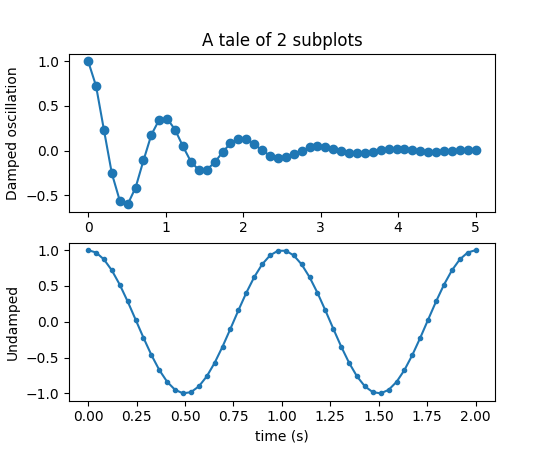## Examples using `matplotlib.pyplot.subplot`¶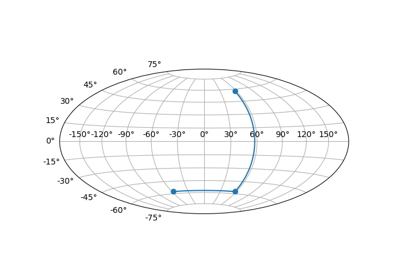Custom projection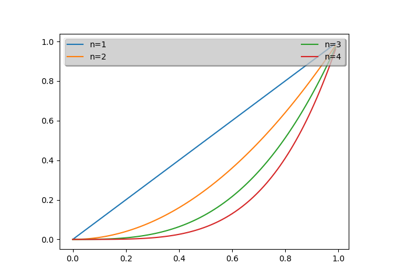Whats New 0.98.4 Legend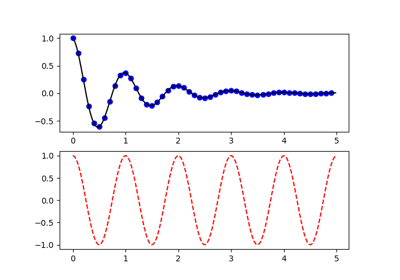Pyplot Two Subplots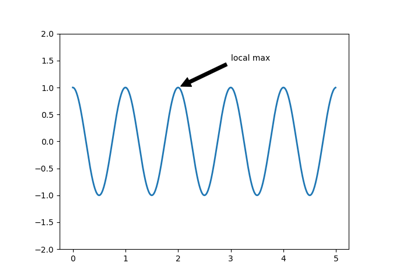Pyplot Annotate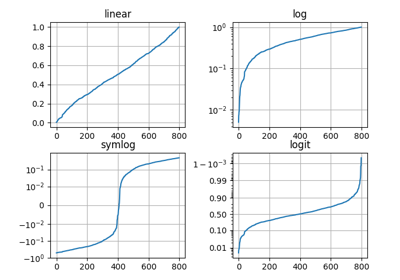Pyplot Scales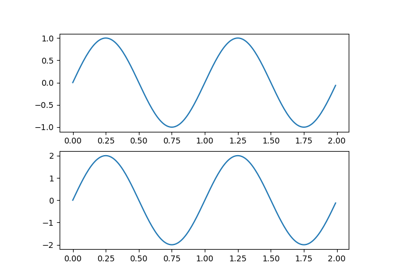Multiple Figs Demo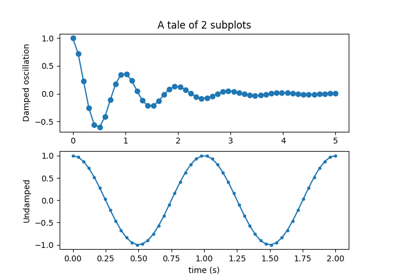Multiple subplots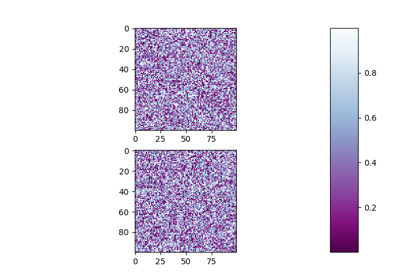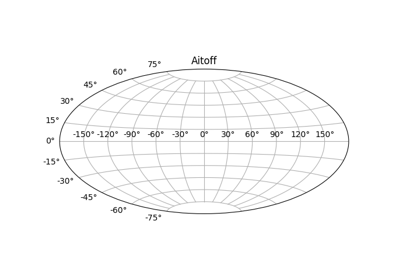Geographic Projections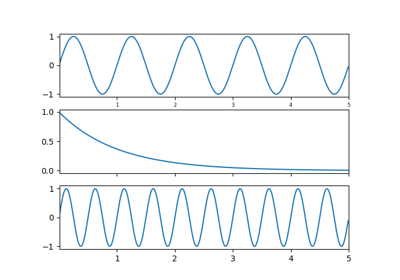Shared Axis Demo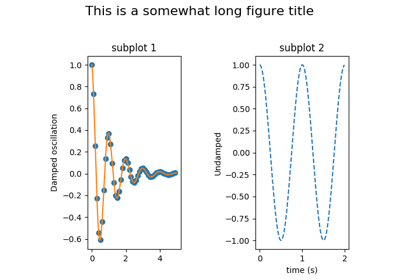Figure Title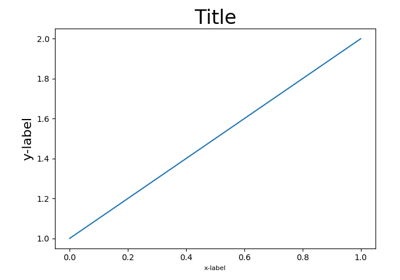Demo Tight Layout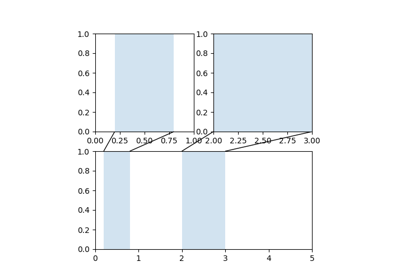Axes Zoom Effect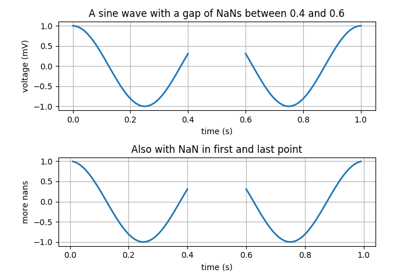Nan Test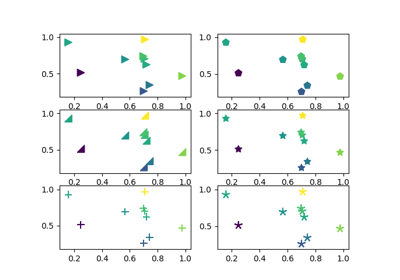Scatter Star Poly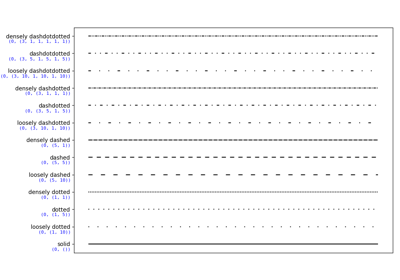Linestyles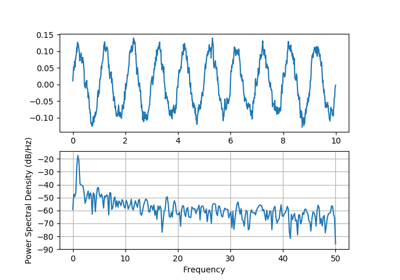Psd Demo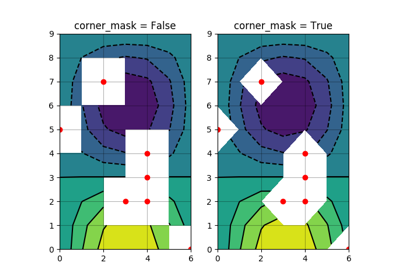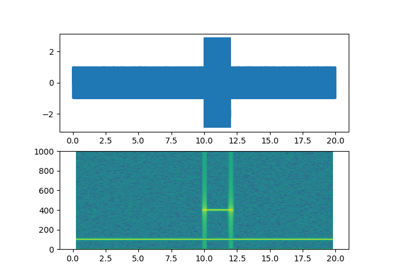Spectrogram Demo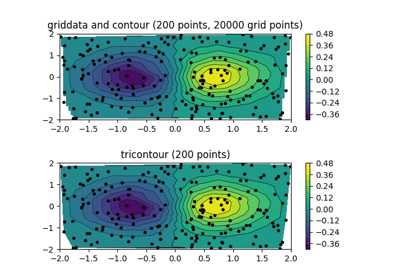Tricontour Vs Griddata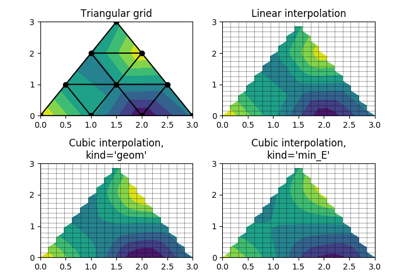Triinterp Demo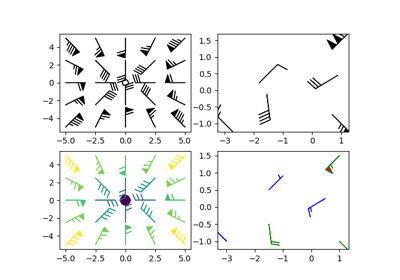Barb Demo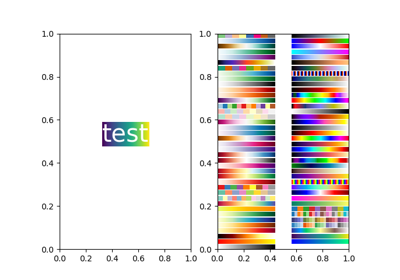Demo BboxImage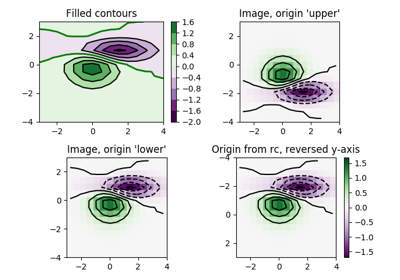Contour Image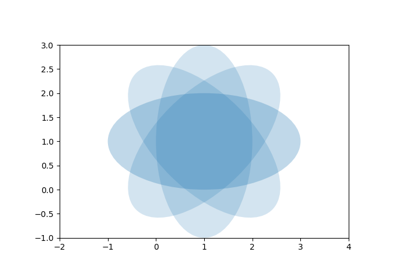Ellipse Rotated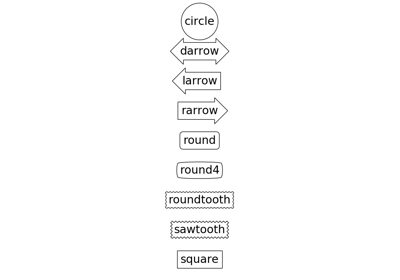Fancybox Demo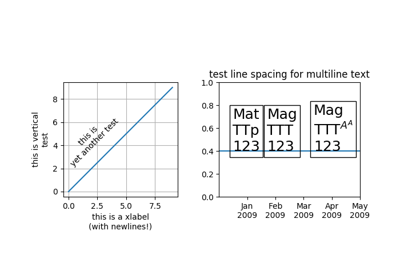Multiline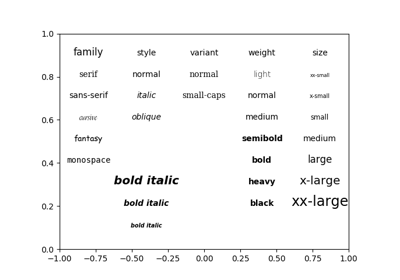Fonts Demo Kw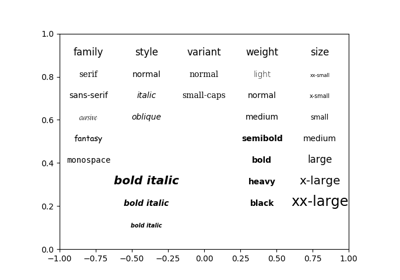Fonts Demo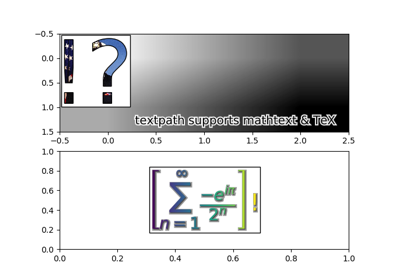Demo Text Path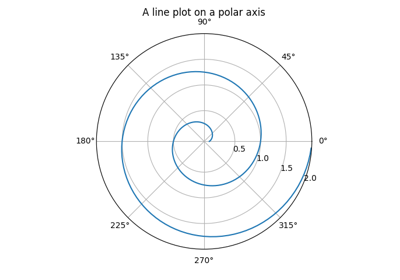Polar Demo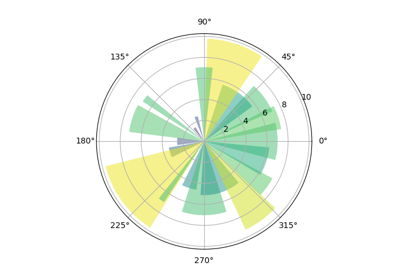Pie chart on polar axis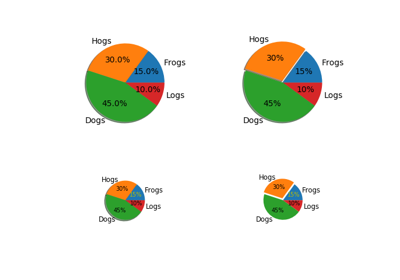Pie Demo2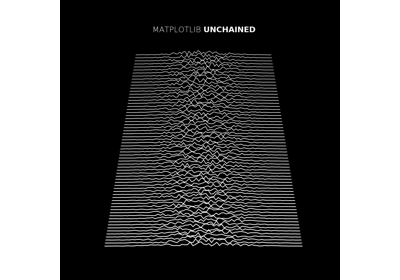MATPLOTLIB UNCHAINED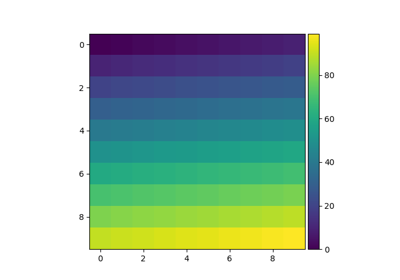Simple Colorbar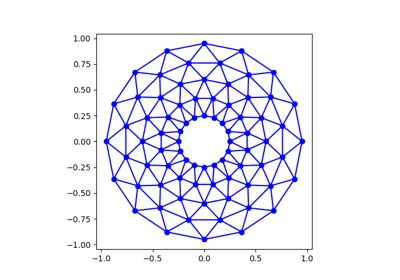Trifinder Event Demo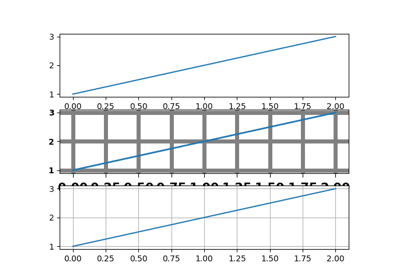Customize Rc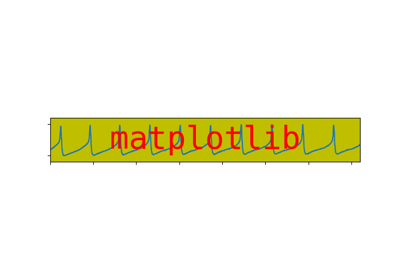Logo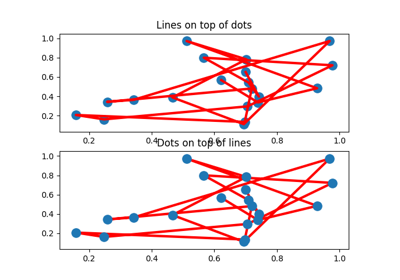Zorder Demo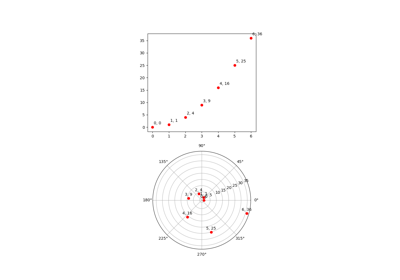Transoffset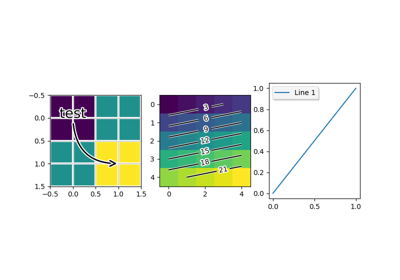Patheffect Demo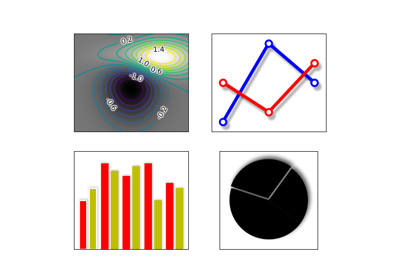Demo Agg Filter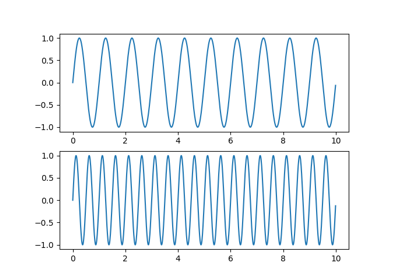Sharing axis limits and views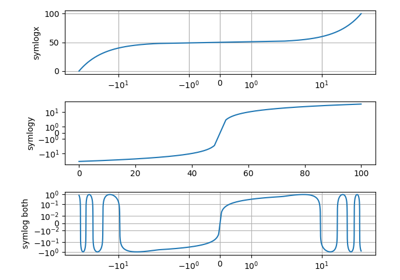Symlog Demo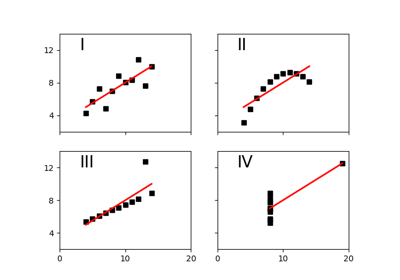Anscombe’s Quartet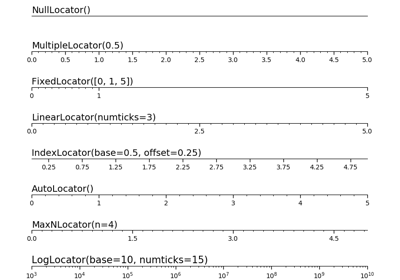Tick locators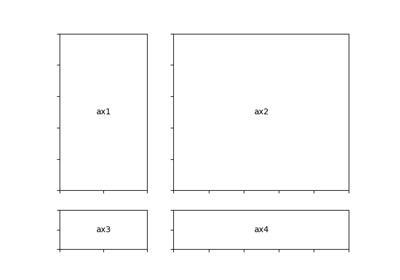Demo Gridspec05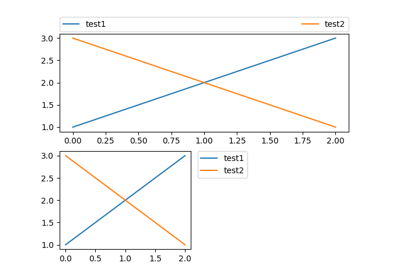Simple Legend01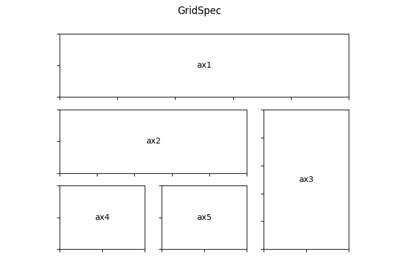Demo Gridspec02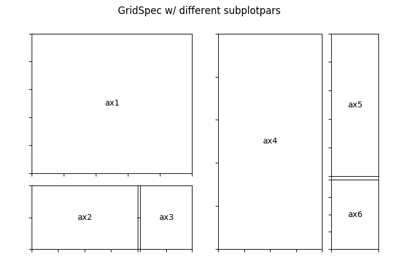Demo Gridspec03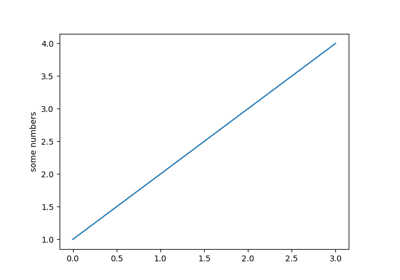Pyplot tutorial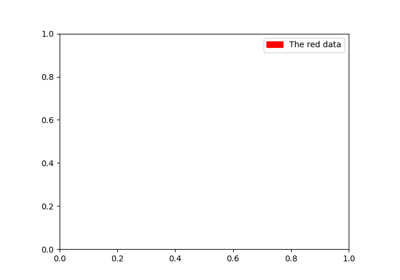Legend guide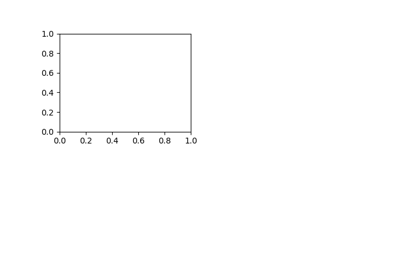Customizing Location of Subplot Using GridSpec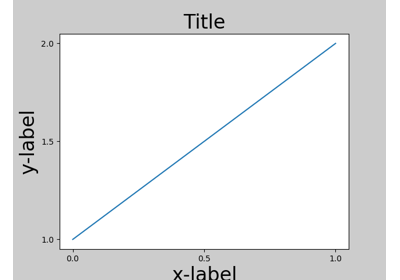Tight Layout guide